# Uncertainty in Physical Measurements Module 5 Student Guide

Uncertainty Module 5 - UM Student Guide April 25, 2016, 2:56 p.m.

## Data with Two Variables

Often data have two variables, such as the magnitude of the force F exerted on an object and the object’s acceleration a. In this Module we will examine some ways to determine how one of the variables, such as the acceleration, depends on the other variable, such as the force.

Say we have collected data for the acceleration a of a cart of mass M for force F with the apparatus of Figure 1. We assume that the mass of the string and the pulley are negligible, and the pulley is frictionless. Then the force F exerted on the cart is equal to mg, where g is the acceleration due to gravity. We collect data for a number of different masses m.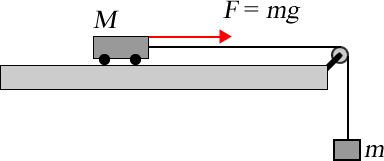Figure 1

We want to determine how the acceleration depends on the force. The acceleration is some function of the force:

$$a=f(F)$$                (1)

In this case the variable F is called the independent variable, it is the quantity that is being experimentally changed by changing the mass m. Then the variable a is called the dependent variable, and its value depends on the value of the independent variable.

Table 1 shows the data for the experiment. The uncertainties were found using the techniques we learned about in Uncertainty Module 2 and Uncertainty Module 3.

 F (N) a (m s-2) $$0.25 \pm 0.03$$ $$0.6 \pm 0.1$$ $$0.74 \pm 0.03$$ $$1.4 \pm 0.1$$ $$1.23 \pm 0.03$$ $$2.4 \pm 0.2$$ $$1.72 \pm 0.03$$ $$3.4 \pm 0.3$$

Table 1

## Graphing the Data

There is probably no better way to explore data than with a graph. Figure 2 shows a graph of the point:

$$(F_1, a_1) = (0.25 \pm 0.03, 0.5 \pm 0.1)$$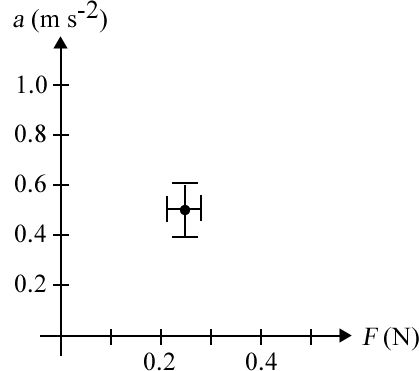Figure 2

The dot is at the values of the force and acceleration, and the length of the bars through the dot indicate the values of the uncertainties.

Sometimes the uncertainty in one of the two variables is zero or negligible. As an example, consider the temperature measurements we thought about in Uncertainty Module 2. We wish to calibrate the digital thermometer that reads temperatures to the tenth of a degree with the better thermometer that reads temperatures to the hundredths of a degree. So for a number of different temperatures we measure using both instruments. There is an uncertainty in our measurements of the temperature with the better instrument, but there is no uncertainty in the reading of the less good instrument: it reads, say, exactly 12.8 when we measure the temperature with the better instrument to be $$12.820 \pm 0.006\ ^\circ {\rm C}$$ . Then the plot of the datapoint: $$(reading, t)=(12.8,12.820 \pm 0.006)$$is shown in Figure 3.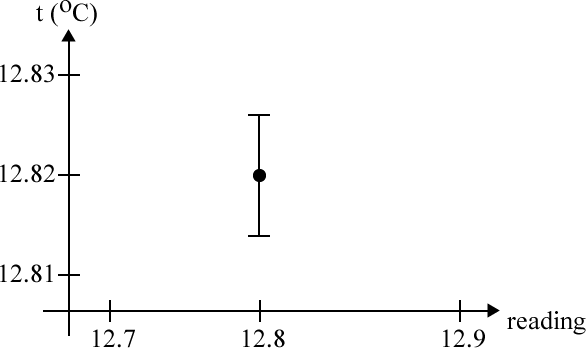Figure 3If there is only a significant uncertainty in one of the variables, as in Figure 3, that uncertainty must be in the dependent variable, i.e. the one that is graphed on the vertical axis. The data in Table 1 have been plotted in a Printable Version (PDF) of Figure 10 which we suggest you print.

##Activity 1

If we assume Newton’s 2nd Law is correct for the data, then for a frictionless cart:

$$a= {1 \over M}F$$                    (2)

Eqn. 2 is called a model of the physical system of Figure 1. From the equation, the slope of a straight line through the data points is equal to 1/M.

Draw the best straight line that you can through all the data points. You have a “free” data point at the origin, since the acceleration is exactly zero when there is no applied force. Considering that the uncertainties in the values of the data are saying that the experimenter believes that the actual value is probably within the range given by the uncertainties, does the line have to go through all of the rectangles defined by the uncertainties or only most of them? Explain.

If the uncertainties in the data are based on a triangular probability distribution, what is a reasonable numerical value for the word “most”? What about for a Gaussian probability distribution function?

Find the slope m of the line. What are the units of the slope?

Calculate M. What are its units?

In finding the “best” straight line, you may have noticed that you can wiggle the ruler around a bit and still account pretty well for the data within the experimental uncertainties. Determine how much you can wiggle the ruler and still account for the data. Does the line with the maximum or the minimum slope have to go through all the bars representing the uncertainty, or only most of them? Explain.

The amount of wiggle you can do with the ruler and still account for the data determines the uncertainty in the value of slope. Determine what that uncertainty is.

Finally, present your experimental determination of M including its uncertainty. Recall from Uncertainty Module 4 that if a quantity is raised to a power, $$z=x^n$$, then the uncertainty in z is given by $$u(z) = |n x^{(n-1)}u(x)|$$ . Here $$M=m^{-1}$$ , i.e. n = –1.

You will want to staple Figure 10 with the lines you have drawn on it into your notebook.

##Question 1

Measuring the temperature t and pressure p of a fixed volume of gas and extrapolating the data to when the pressure is zero can find the value of the absolute zero. Figures 4a and 4b show some student-collected data of such an experiment as reported by Taylor [J.R. Taylor, An Introduction to Error Analysis (University Science Books, Mill Valley CA, 1982), 160].  Which plot should be used to determine the value of absolute zero? Why? Note that you are not being asked to do the determination, although of course you may do so if you wish.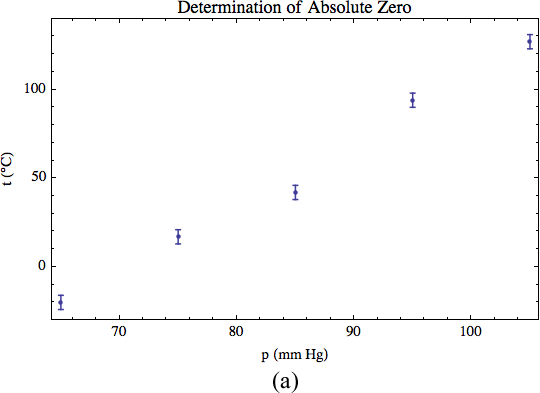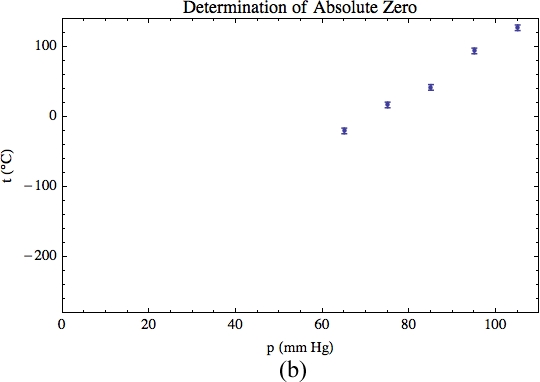Figure 4You may be used to writing Newton’s 2nd Law as:

F = Ma              (3)

Although algebraically this is identical to the form we used in Eqn. 2, in terms of communicating the relationship between forces and acceleration it is misleading. Eqn. 3 implies that the independent variable is a which causes forces F. This is clearly not correct. Eqn. 2, then, best expresses the relation between force and acceleration: forces cause accelerations.

## Least-Squares Fitting

In Activity 1 you fit the data of Table 1 to a model by hand. The model was given by Eqn. 2, and you used the graph of the data to perform the fit and determine the value and uncertainty in the parameter 1/M.

Often we use computers to do such fits numerically. The most common type of fitting is to a polynomial model:

\begin{align*} y &= a_0 + a_1x + a_2 x^2 + ...\\ &= \sum_{i=0}^N a_i x^i \end{align*}               (4)

For example, if the model is a straight line, y = mx + b, then a0 is the intercept b, a1 is the slope m, and all other of the parameters aj are zero. If the model is a parabola, y = c x2, then the only non-zero parameter is a2 which is c in the model. In general, the fit determines the values of the parameters ak that are non-zero.

Say we are fitting to an arbitrary model:

y = f (x)           (5)

We have a series of values of the data: $$(x_1,y_1),(x_2,y_2),...,(x_N,y_N)$$ . For each datapoint, the fitted value of the dependent variable, yi,fit, is given by:

yi,fit = f (xi)           (6)

However, the experimental value of yi is unlikely to be exactly equal to yi,fit. We define the residual ri to be:

\begin{align*} r_i &= y_i - y_{i, {\rm fit}}\\ &= y_i - f(x_i) \end{align*}               (7)

Just as for the deviations we learned about in Uncertainty Module 1, for a perfect fit the sum of the residuals for all the data is zero. However, again similar to the deviations in Module 1, the sum of the squares of the residuals is not zero. It is a measure of how much the model differs from the data.

The most common technique for computer fitting of data to a model is called least-squares. The name is because it finds the values of the fitted parameters for which the sum of the squares of the residuals is a minimum.

There is a famous quartet of (x, y) pairs devised by Anscombe [F.J. Anscombe, American Statistician 27 (Feb. 1973), pg. 17]. Here is a listing of a Python program that loads the usual libraries, defines the four datasets, and does some analysis of the first dataset:   Anscombe.py :

from pylab import *
from scipy import *

# Anscombe's first dataset
A1x = [10, 8, 13, 9, 11, 14, 6, 4, 12, 7, 5]
A1y = [8.04, 6.95, 7.58, 8.81, 8.33, 9.96, 7.24, 4.26, 10.84,4.82, 5.68]

# The second
A2x = [10, 8, 13, 9, 11, 14, 6, 4, 12, 7, 5]
A2y = [9.14, 8.14, 8.74, 8.77, 9.26, 8.10, 6.13, 3.10, 9.13, 7.26, 4.74]

# The third
A3x = [10, 8, 13, 9, 11, 14, 6, 4, 12, 7, 5]
A3y = [7.46, 6.77, 12.74, 7.11, 7.81, 8.84, 6.08, 5.39, 8.15, 6.42, 5.73]

# The fourth
A4x = [8, 8, 8, 8, 8, 8, 8, 19, 8, 8, 8]
A4y = [6.58, 5.76, 7.71, 8.84, 8.47, 7.04, 5.25, 12.50, 5.56, 7.91, 6.89]

print "First dataset:"
print "Mean of x:", mean(A1x)
print "Variance of x:", var(A1x, ddof = 1)
print "Mean of y:", mean(A1y)
print "Variance of y:", var(A1y, ddof = 1)
print "Straight line fit:", polyfit(A1x, A1y, 1, full = True)

Note that the first of the four datasets consists of the values of x in A1x, and the values of y in A1y. The other three datasets are similarly named except for the number in the variable name.

The program computes the means and variances of the x and y variables. The last line fits the data to a straight line. Run the program. The output window will look like Figure 5 except for the red boxes and labels, which have been added.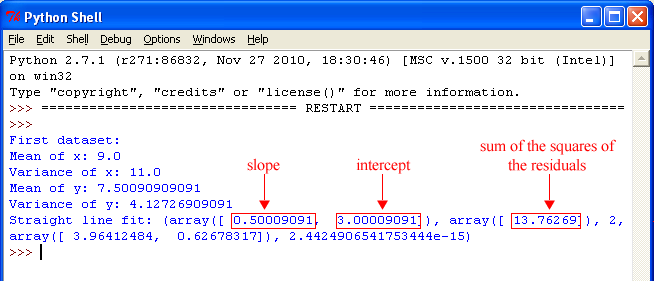Figure 5

There is a lot of information in the results of the fit, but we will concentrate on the slope, intercept, and sum of the squares of the residuals.

##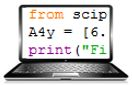Activity 2

Copy and paste the program lines that computes the means, variances, and does the fit and change the copied lines so that the program does the calculation on the 2nd, 3rd, and 4th datasets.

If you just look at the values for the four datasets, i.e. means, variances, and the slopes, intercepts, and the sum of the squares of the residuals of the fit, what might you conclude about whether or not the four datasets are almost identical?

Plot the first dataset with:

plot(A1x, A1y,'bo')
show()

Look at the plot, and then plot the other three datasets. Now what do you think about the similarity of the four datasets? Is a straight line model appropriate for each of the datasets?

What can you conclude about the relative importance of the numbers that characterize the data compared to a graphical exploration of the data?

Imagine we are fitting some data to a straight line: y = mx + b. If there is only one datapoint, then no such fit is possible: any line going through the datapoint is equivalent to any other line going through the datapoint. If there are exactly two datapoints, then there is no doubt about the values of the slope and intercept: they are the slope and intercept of the line connecting the two points. However, if there are three or more datapoints, then we can imagine a range of slopes and intercepts of lines that more-or-less are consistent with the data.

The degrees of freedom of a fit are the number of datapoints minus the number of parameters to which we are fitting, which is two for a straight line. Fits with negative degrees of freedom are impossible. Fits with zero degrees of freedom are exact.

##Question 2

Say you are fitting the data of Table 1 to a straight line with an added parabolic term:

$$a = mF + b + cF^2$$

What are the degrees of freedom of the fit?

## Evaluating the Quality of a Fit

In Activity 2 we learned the graphs are an important tool in evaluating fits. Now we will learn about some quantitative ways of evaluating a fit.

The sum of the squares of the residuals of a fit, ss, is:

\begin{align*} ss = \sum_{i=0}^N [y_i - f(x_i)]^2 \end{align*}               (8)

where we have fit the data to the model y = f(x) and there are N datapoints. It measures the “goodness” of the fit, with smaller values meaning a better fit. But there is no objective way to determine if the value of ss is “small” or “large.”

However, if the data have uncertainties in the dependent variable, $$u(y_i)$$ , then we can weight each residual by 1 over that uncertainty, and form the sum of the squares of the weighted residuals. This sum is called the chi-squared, $$\chi^2$$. ($$\chi$$is the Greek letter “chi” which rhymes with the word “eye.”)

\begin{align*} \chi^2 = \sum_{i=0}^N \left[ {y_i - f(x_i) \over u(y_i)} \right]^2 \end{align*}               (9)

Now a “least-squares” fit finds the minimum in the $$\chi^2$$ , which may be for different values of the fitted parameters than the values found by minimising the sum of the squares of the residuals.

If the data are correct and the model is reasonable, the $$\chi^2$$ should be roughly equal to the number of degrees of freedom. If the  $$\chi^2$$ is much larger than the number of degrees of freedom, the fit is poor. If the $$\chi^2$$ is much less than the number of degrees of freedom, the fit is too good to be true.

Although you have learned in these Modules that almost all numbers used to characterize the physical world have uncertainties, sometimes the value of those uncertainties is not given. If the value of an uncertainty is given, such as in  $$x = 3 \pm 1$$ , we say that the uncertainty is explicit.

##Questions

1. You are fitting data to some model. The data includes explicit uncertainties only in the dependent variable. The $$\chi^2$$ divided by the number of degrees of freedom is 0.05. If the model is appropriate for the data, and the experimental values of the independent and dependent variables are correct, what is probably wrong?
2. You are fitting data to some model. The data includes explicit uncertainties only in the dependent variable. The $$\chi^2$$ divided by the number of degrees of freedom is 14. If the data, including the uncertainties, are correct, what is probably wrong?

Although almost all real experimental data have uncertainties in at least the dependent variable, many standard least-squares fitters are not capable of dealing with those uncertainties. This means that for analysis of experimental data they are often not good enough. On the Practical computers, the Polynomial Fit program in the LabVIEW Shortcuts folder does allow for data with uncertainties in one or both of the variables in the dataset.

Often real experimental data, such as in Table 1, has uncertainties in the independent variable. The standard way of dealing with this case is called the effective variance method. Say we are fitting the data to y = f (x) and have a datapoint with a value of the independent variable x and an uncertainty ux. Then we can form an effective uncertainty in the value of the dependent variable, ueff, due to the uncertainty in x as shown in Figure 6.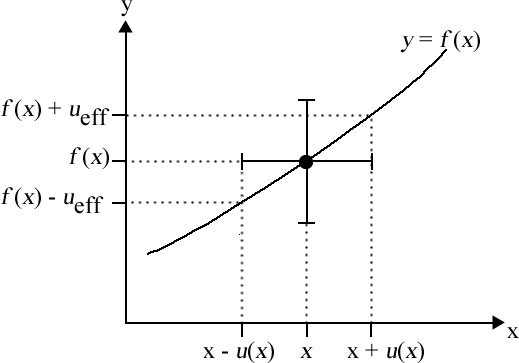Figure 6

Then the total uncertainty in the y coordinate is the uncertainty in y, u(y), combined with the effective uncertainty due to u(x) in quadrature.

$$u_{total}(y) = \sqrt{u(y)^2 + u_{eff}^2}$$                (10)

Then utotal is used in the fit the same way that u(y) is used for data with explicit uncertainties only in the dependent variable.

## An Example

A thermocouple is a device that emits a voltage that depends on its temperature. Thermocouples are often used as thermometers. Figure 7 shows some student-collected data on calibrating a thermocouple that was presented by Bevington [Philip R. Bevington, Data Reduction and Analysis (McGraw-Hill, 1969), pg. 138]. The student assigned an uncertainty to the voltage, but not to the temperature. Also shown in the figure is the result of fitting the data to a straight line. The results of the fit were:

slope:  $$0.0412 \pm 0.0004$$

intercept:   $$-0.98 \pm 0.02$$

chi-squared: 21.05

degrees of freedom: 19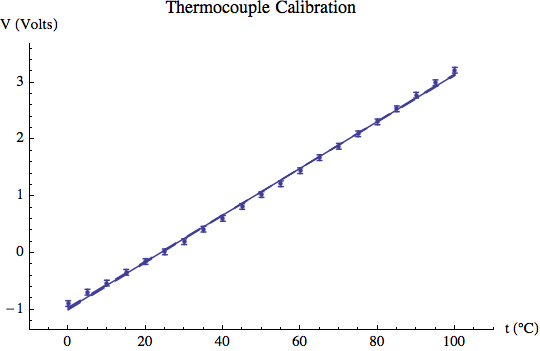Figure 7

##Questions

1. Just from the numerical results of the fit and from Figure 7, is this a good fit to a reasonable model?
2. Figure 8 shows a plot of the residuals. Now what do you think of the fit?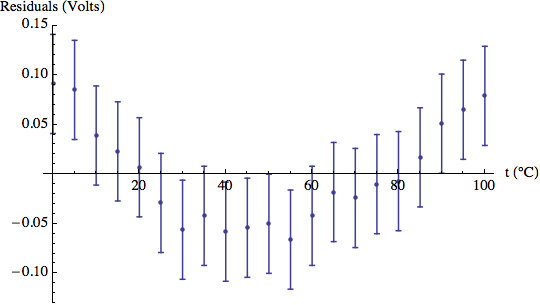Figure 8

1. We add a quadratic term to the fit, so we are fitting to: V = mt + b + ct2. The numerical results of the fit are:

slope: $$m = 0.035 \pm 0.001$$
intercept: $$b = -0.89 \pm 0.03$$
quadratic term: $$c = -.00006 \pm 0.00001$$
chi-squared: 1.007
degrees of freedom: 18

The graphical result of the fit including a plot of the residuals as an insert is shown in Figure 9. Is this a good fit? Are there any problems with it? If so, what are they and how can they be explained?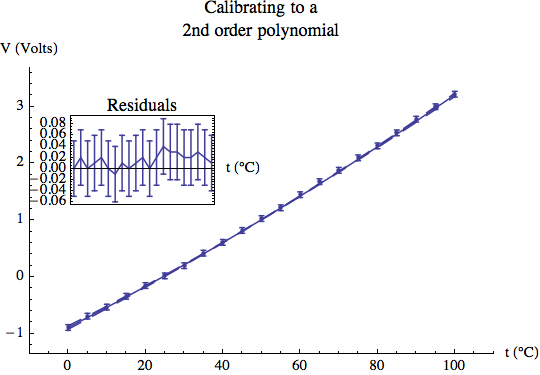Figure 9

We began this Module using a theory, Newton’s 2nd Law, as a model for the force-acceleration data. For the thermocouple calibration, we have not used any theory: we have let the data “talk to us” to determine an appropriate model for the relation between temperature and voltage.

## Summary of Names, Symbols, and Formulae

independent variable: the quantity that is varied in an experiment

dependent variable: the quantity whose value changes because of changes in the independent variable

model: some formula or relation that represents a physical system

residual r : the fitted value of the dependent variable minus the experimental value of the dependent variable

least squares: a fitting technique that minimizes the sum of the squares of the residuals

degrees of freedom: the number of datapoints minus the number of parameters to which the data are being fit

chi-squared $$\chi^2$$ : the sum of the squares of the residuals each divided by the uncertainty

effective variance method: a technique to account for uncertainties in the dependent variable of a dataset

explicit uncertainty: the value of the uncertainty in some quantity is explicitly given

This Guide was written by David M. Harrison, Dept. of Physics, Univ. of Toronto, September 2013.